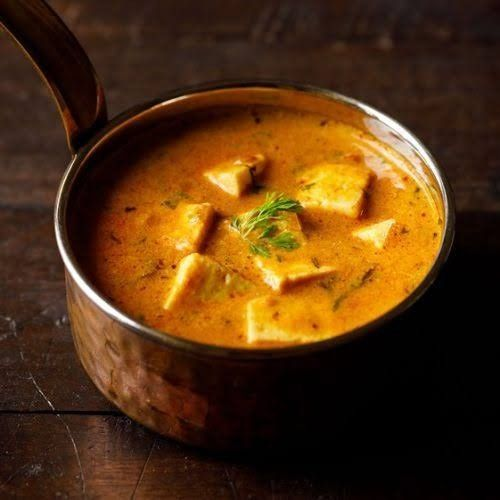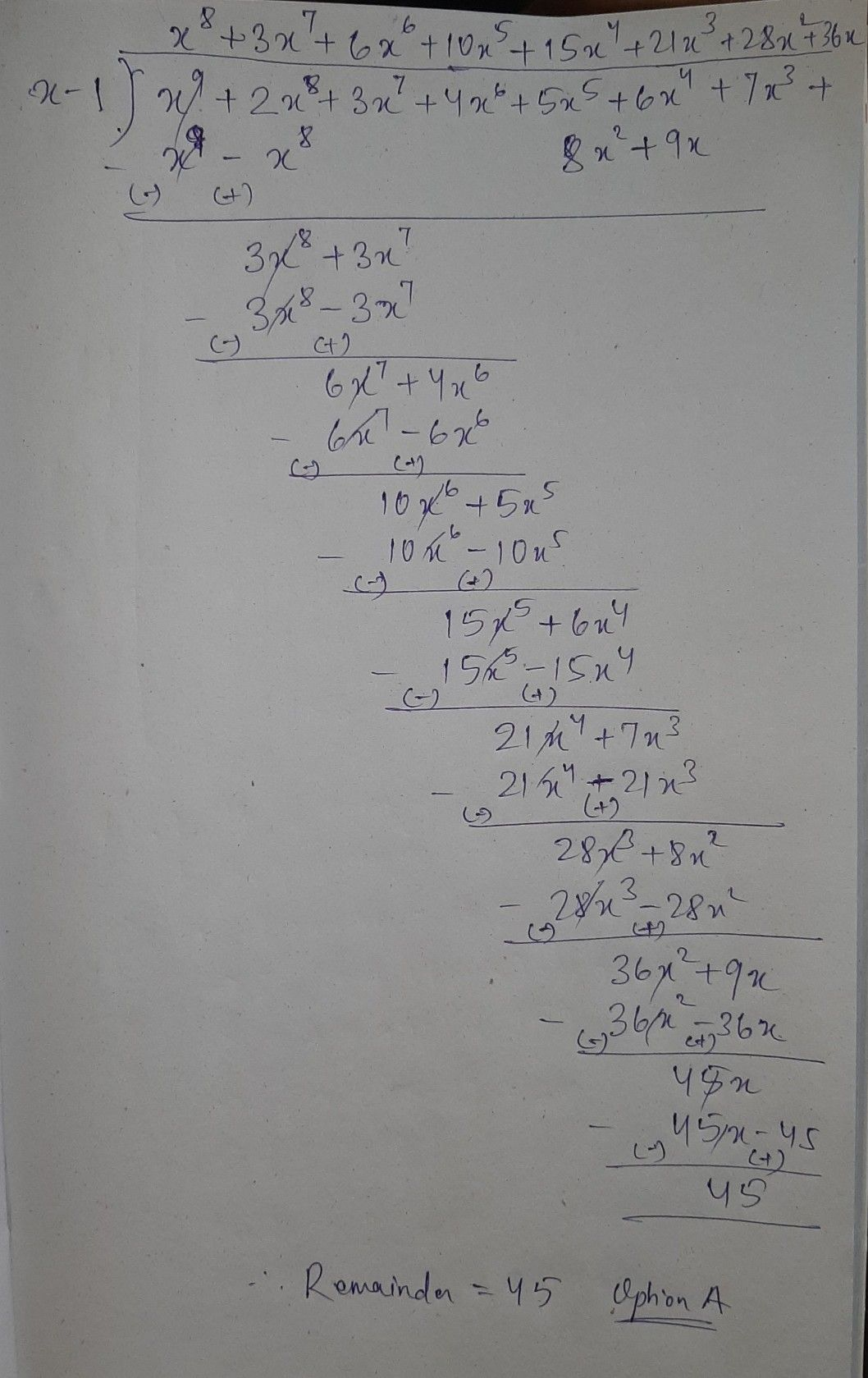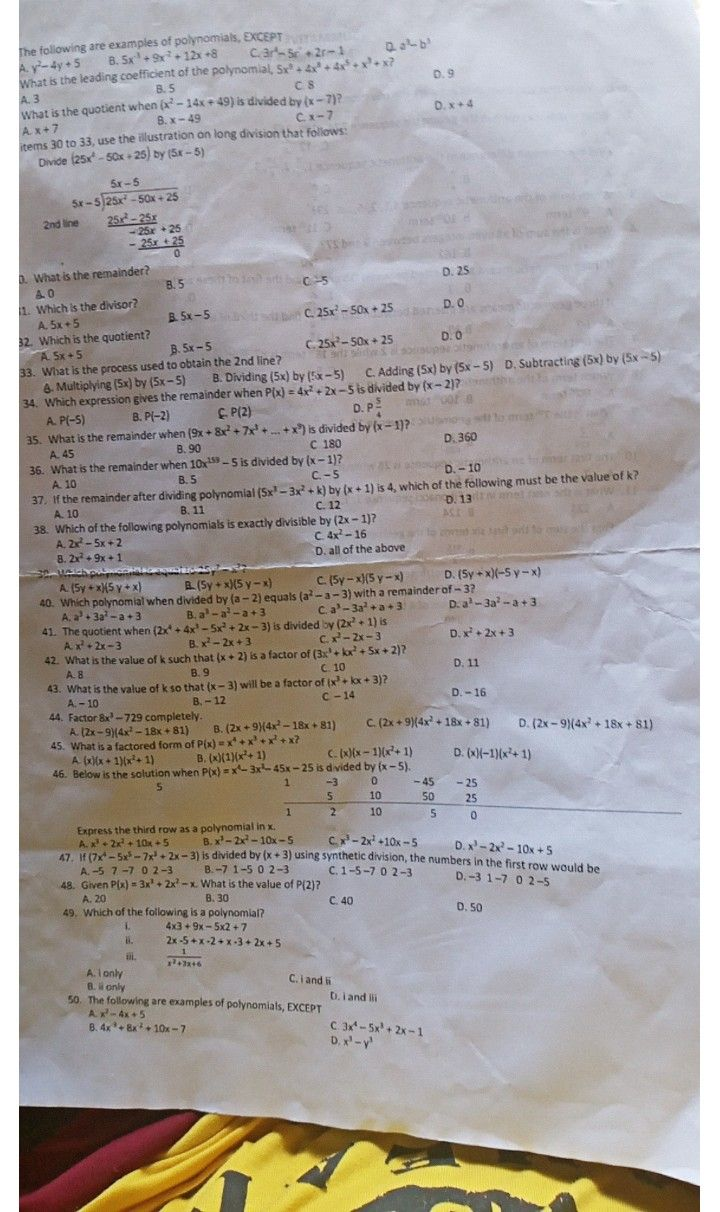Symbol
Problem$12\right)$ $4$ $35.1$ What is the remainder when $\left(9x+8x^{2}+7x^{3}+...4x\right)$ is divided by $\left(x-1\right)7$ A. $45$ B. $90$ $C$ $120$ D. $250$ $36$ What is the remainder when $10x^{13}-5$ is divided by $y\left(x-1\right)7$
10th-13th grade
Geometry
Search count: 105
SolutionQanda teacher - BhanuStudent
salamatt poQanda teacher - Bhanu
please evaluate the answer with 5 stars and like my profile and if possible gift some coinsStudent